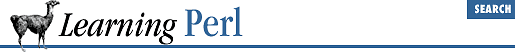home | O'Reilly's CD bookshelfs | FreeBSD | Linux | Cisco | Cisco Exam

### A.15 Chapter 16, System Database Access

1. Here's one way to do that:

```\$: = " ";
while (@pw =

getpwent) {
(\$user, \$gid, \$gcos) =

@pw[0,3,6];
(\$real) = split /,/, \$gcos;
\$real{\$user} = \$real;
\$members{\$gid} .= " \$user";
(\$last) = (split /\s+/, \$real)[-1];
\$last{\$user} = "\L\$last";
}

while (@gr = getgrent) {
(\$gname,\$gid,\$members) = @gr[0,2,3];
\$members{\$gid} .= " \$members";
\$gname{\$gid} = \$gname;
}

for \$gid (

sort by_gname keys %gname) {
%all = ();
for (split(/\s+/, \$members{\$gid})) {
\$all{\$_}++ if

length \$_;
}
@members = ();

foreach (sort by_last keys %all) {

push(@members, "\$real{\$_} (\$_)");
}
\$memberlist =

join(", ", @members);

write;
}

sub by_gname { \$gname{\$a}

cmp \$gname{\$b}; }
sub by_last { (\$last{\$a} cmp \$last{\$b})

|| (\$a cmp \$b); }

format STDOUT =
@<<<<<<<< @<<<<<<<< ^<<<<<<<<<<<<<<<<<<<<<<<<<<<<<<<<<<<<<
\$gname{\$gid}, "(\$gid)", \$memberlist
~~                  ^<<<<<<<<<<<<<<<<<<<<<<<<<<<<<<<<<<<<<
\$memberlist
.```

Yes, this one needs some explaining.A.14 Chapter 15, Other Data TransformationA.16 Chapter 17, User Database Manipulation